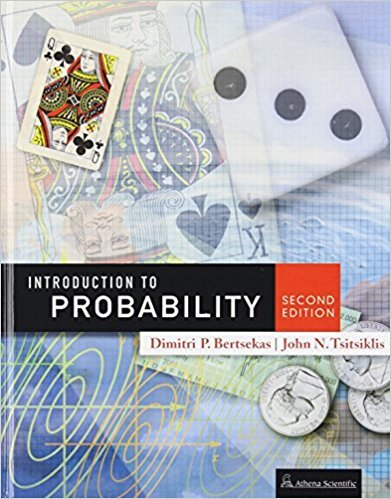×
×

# Solutions for Chapter 9: Classical Statistical Inference## Full solutions for Introduction to Probability, | 2nd Edition

ISBN: 9781886529236Solutions for Chapter 9: Classical Statistical Inference

Solutions for Chapter 9
4 5 0 384 Reviews
14
1
##### ISBN: 9781886529236

This expansive textbook survival guide covers the following chapters and their solutions. Since 30 problems in chapter 9: Classical Statistical Inference have been answered, more than 8529 students have viewed full step-by-step solutions from this chapter. Introduction to Probability, was written by and is associated to the ISBN: 9781886529236. Chapter 9: Classical Statistical Inference includes 30 full step-by-step solutions. This textbook survival guide was created for the textbook: Introduction to Probability,, edition: 2.

Key Statistics Terms and definitions covered in this textbook
• 2 k p - factorial experiment

A fractional factorial experiment with k factors tested in a 2 ? p fraction with all factors tested at only two levels (settings) each

A formula used to determine the probability of the union of two (or more) events from the probabilities of the events and their intersection(s).

A variation of the R 2 statistic that compensates for the number of parameters in a regression model. Essentially, the adjustment is a penalty for increasing the number of parameters in the model. Alias. In a fractional factorial experiment when certain factor effects cannot be estimated uniquely, they are said to be aliased.

• Alternative hypothesis

In statistical hypothesis testing, this is a hypothesis other than the one that is being tested. The alternative hypothesis contains feasible conditions, whereas the null hypothesis speciies conditions that are under test

• Average

See Arithmetic mean.

• Bayes’ theorem

An equation for a conditional probability such as PA B ( | ) in terms of the reverse conditional probability PB A ( | ).

• Biased estimator

Unbiased estimator.

• Coeficient of determination

See R 2 .

• Comparative experiment

An experiment in which the treatments (experimental conditions) that are to be studied are included in the experiment. The data from the experiment are used to evaluate the treatments.

• Completely randomized design (or experiment)

A type of experimental design in which the treatments or design factors are assigned to the experimental units in a random manner. In designed experiments, a completely randomized design results from running all of the treatment combinations in random order.

• Conidence level

Another term for the conidence coeficient.

• Continuous distribution

A probability distribution for a continuous random variable.

• Contour plot

A two-dimensional graphic used for a bivariate probability density function that displays curves for which the probability density function is constant.

• Correction factor

A term used for the quantity ( / )( ) 1 1 2 n xi i n ? = that is subtracted from xi i n 2 ? =1 to give the corrected sum of squares deined as (/ ) ( ) 1 1 2 n xx i x i n ? = i ? . The correction factor can also be written as nx 2 .

• Critical region

In hypothesis testing, this is the portion of the sample space of a test statistic that will lead to rejection of the null hypothesis.

• Cumulative distribution function

For a random variable X, the function of X deined as PX x ( ) ? that is used to specify the probability distribution.

• Dependent variable

The response variable in regression or a designed experiment.

• Distribution free method(s)

Any method of inference (hypothesis testing or conidence interval construction) that does not depend on the form of the underlying distribution of the observations. Sometimes called nonparametric method(s).

• Error sum of squares

In analysis of variance, this is the portion of total variability that is due to the random component in the data. It is usually based on replication of observations at certain treatment combinations in the experiment. It is sometimes called the residual sum of squares, although this is really a better term to use only when the sum of squares is based on the remnants of a model-itting process and not on replication.

• Experiment

A series of tests in which changes are made to the system under study

×